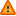FAQ/rankit - CBU statistics WikiYou should trust both wikis because the password could be read by the particular administrators.

Clear message
location: FAQ / rankit

# Rankit and percentage-bend correlations (for downweighting outliers)

The rankit correlation is easily computed in any statistical package using a transform of the two variables being correlated and has been recommended by Bishara and Hittner (2012) when correlating data that is assymmetric or heavy tailed. They conclude that

"With most sample sizes (n > 20), Type I and Type II error rates were minimized by transforming the data to a normal shape prior to assessing the Pearson correlation. Among transformation approaches, a general purpose rank-based inverse normal transformation (i.e., transformation to rankit scores) was most beneficial. However, when samples were both small (n < 10) and extremely nonnormal, a permutation test often outperformed other alternatives, including various bootstrap tests."

The rankit transformation for x is of form

rankit(x) = $$\mbox{INV.NORMAL}(\frac{x - 0.5){n})$$

Wilcox (1994) also suggested an alternative, the percentile-bend correlation, to the Pearson correlation which downweights a specified percentage of observations furthest from the median and is consequently less sensitive to outliers than the Pearson correlation. Pernet, Wilcox and Rousselet (2012) further found that a 20% percentage-bend correlation gave better results than Pearson’s or Spearman’s correlations. The 20% percentage-bend correlation is computed and tested using this spreadsheet. This spreadsheet could also be used to obtain bootstrap confidence intervals. Wilcox (2012, page 446) comments that the Pearson and percentile-bend give similar values for normal distributions.

The percentile-bend correlation can be run in R and is available in the file Rallfun-v20.R written by Rand Wilcox (which is downloadable from here) and can be added into a folder such as the R library (default folder) and into a R session via the source command. The pbcor function produces the correlation and the results of a significance test (using the same t statistic on n-2 df, as for the Pearson as recommended by Wilcox (2012)) whilst the corb function produces a 95% confidence interval.

source("Rallfun-v20.R")
x <- c(2,3,1,2)
y <- c(1,5,4,3)
pbcor(x,y,beta=0.2)
corb(x,y,nboot=599)

References

Bishara AJ and Hittner JB (2012) Testing the Significance of a Correlation With Nonnormal Data: Comparison of Pearson, Spearman, Transformation, and Resampling Approaches. Psychological Methods 17(3) 399-417. Note than a Pdf copy of this paper may be downloaded for free by CBSU users using the Psychnet weblink.

Pernet CR, Wilcox R and Rousselet GA (2012) Robust Correlation Analyses: False Positive and Power Validation Using a New Open Source Matlab Toolbox. Front Psychol. 3 606.

Wilcox RR (1994) The percentage bend correlation coefficient. Psychometrika 59 601–616.

Wilcox RR (2012) Introduction to robust estimation and hypothesis testing. Third edition. San Diego, CA:Elsevier.